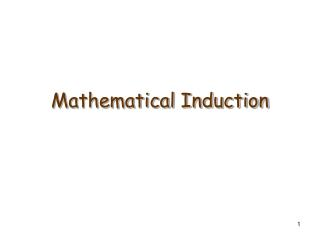DownloadDownload PresentationMathematical Induction

# Mathematical Induction

Télécharger la présentation## Mathematical Induction

- - - - - - - - - - - - - - - - - - - - - - - - - - - E N D - - - - - - - - - - - - - - - - - - - - - - - - - - -
##### Presentation Transcript

1. Mathematical Induction

2. Mathematical Induction: Example • Show that any postage of ≥ 8¢ can be obtained using 3¢ and 5¢ stamps. • First check for a few particular values: 8¢ = 3¢ + 5¢ 9¢ = 3¢ + 3¢ + 3¢ 10¢ = 5¢ + 5¢ 11¢ = 5¢ + 3¢ + 3¢ 12¢ = 3¢ + 3¢ + 3¢ + 3¢ • How to generalize this?

3. Mathematical Induction: Example • Let P(n) be the sentence “n cents postage can be obtained using 3¢ and 5¢ stamps”. • Want to show that “P(k) is true” implies “P(k+1) is true” for any k ≥ 8¢. • 2 cases: 1) P(k) is true and the k cents contain at least one 5¢. 2) P(k) is true and the k cents do not contain any 5¢.

4. Mathematical Induction: Example • Case 1:k cents contain at least one 5¢ coin. • Case 2: k cents do not contain any 5¢ coin. Then there are at least three 3¢ coins. Replace 5¢ coin by two 3¢ coins k cents k+1 cents 5¢ 3¢ 3¢ Replace three 3¢ coins by two 5¢ coins k cents k+1 cents 3¢ 3¢ 3¢ 5¢ 5¢

5. Domino Effect • Mathematical induction works like domino effect: • Let P(n) be “The nth domino falls backward”. • If (a) “P(1) is true”; (b) “P(k) is true” implies “P(k+1) is true” Then P(n) is true for every n

6. Principle of Mathematical Induction Let P(n) be a predicate defined for integers n. Suppose the following statements are true: 1.Basis step: P(a) is true for some fixed aZ . 2.Inductive step: For all integers k ≥ a, if P(k) is true then P(k+1) is true. Then for all integers n ≥ a, P(n) is true.

7. Example: Sum of Odd Integers • Proposition:1 + 3 + … + (2n-1) = n2 for all integers n≥1. • Proof (by induction): 1) Basis step: The statement is truefor n=1: 1=12. 2) Inductive step: Assume the statement is true for some k≥1 (inductive hypothesis) , show that it is true for k+1 .

8. Example: Sum of Odd Integers • Proof (cont.): The statement is truefork: 1+3+…+(2k-1) = k2 (1) We need to show it for k+1: 1+3+…+(2(k+1)-1) = (k+1)2 (2) Showing (2): 1+3+…+(2(k+1)-1) = 1+3+…+(2k+1) = 1+3+…+(2k-1)+(2k+1) = k2+(2k+1) = (k+1)2 . We proved the basis and inductive steps, so we conclude that the given statement true. ■ by (1)

9. Important theorems proved by mathematical induction • Theorem 1 (Sum of the first n integers): For all integers n≥1, • Theorem 2 (Sum of a geometric sequence): For any real number r except 1, and any integer n≥0,

10. Example (of sum of the first n integers). • In a round-robin tournament each of the n teams plays every other team exactly once. What is the total number of games played? • 2 ways to solve: 1) Each of the n teams plays n-1 games; this gives a total of n(n-1) games. But each game was counted exactly twice; Thus, the total number of games is n(n-1)/2.

11. Example (of sum of the first n integers). 2) Team 1 plays n-1 games; Team 2 plays n-2 games (not counting the game with team 1); Team 3 plays n-3 games (not counting the games with teams 1 and 2); …. Team n-1 plays 1 game with team n (not including the games counted before). Thus, the total number of games is 1+2+…+(n-2)+(n-1) = n(n-1)/2by Theorem 1.